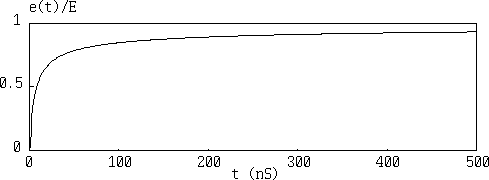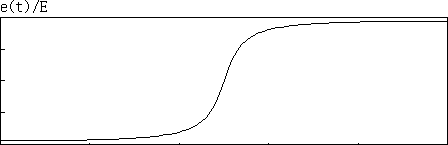# Pulse response of cablesHere is a wave form of step pulse response when a 100 meter long coaxial cable (JIS C3501, 50 Ohm, 3.0 mm insulation diameter) is used. The wave form that rises up steeply first and then rises moderately is often seen as an exponential wave form of C-R charging circuits. However, since in transmission theory of electrical engineering, a condition that a secondary parameter under high frequency of cables is R << w*L, G << w*C holds good, the following approximation is very accurate (1).

```  a = R/Z0/2 + G*Z0/2
b = sqrt(L*C)

where
a= Attenuation constant (neper/m)
L = Inductance (H/m)
C = Capacitance (F/m)
R = Resistance (ohm/m)
G = Conductance (S/m)
G = Conductance (S/m)
Z0 = Characteristic impedance (ohm)
```
On the other hand, R has a big frequency dependency, and under high frequency it increases in proportion to a square root of frequency. However, as long as high quality dielectric material is used, C, L, and G don't have frequency dependency. It means there is no phase distortion in high frequency cables and only attenuation distortion exists. This fact can be confirmed by simple measurement with network analyzer or similar equipments. Therefore transmission theory doesn't have any fundamental error.

However if this is true, since attenuation distortion simply makes wave form dull as you can see from linear circuit theory, the first part and the last part of wave form should be symmetrical. It is impossible that the first part is steep and the last is moderate. It should be like the one shown below.Now you see there is a contradiction between transmission theory and circuit theory, which are supposed to be true in electric engineering. What is going on here? This is the question of the puzzle.

A long time ago I've asked this question to several people but never got a clear answer. People who gave me some kind of answer told me "It is an exponential wave form of C by R of a cable." But please think about it. It is a key of transmission theory that it is unreasonable to think about primary constants such as R, L, C, and G in high frequency cables, so think with secondary constants based on undulation. This denies trustworthy transmission theory. If so, where is a truth?

```  a + j*b = sqrt((R + j*w*L)*(G + j*w*C))

where
j = sqrt(-1)